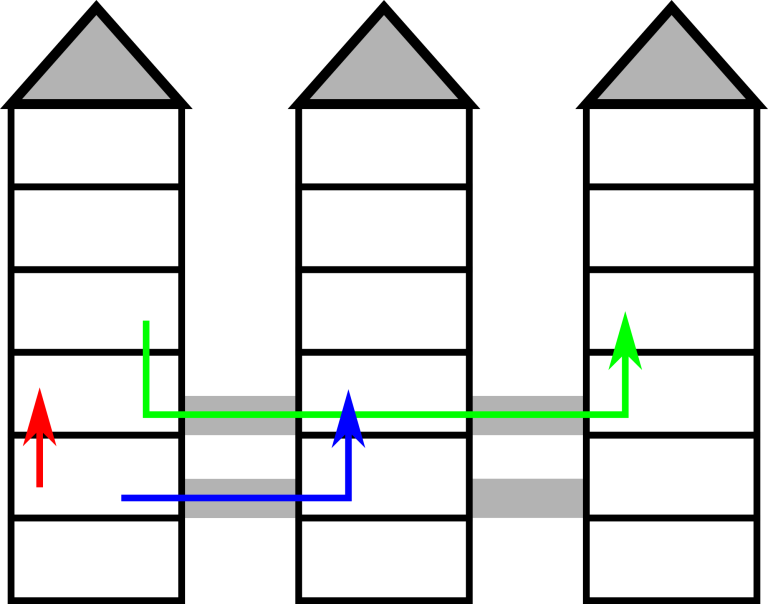# CF1020A New Building for SIS

• 74通过
• 133提交
• 题目来源
• 评测方式 RemoteJudge
• 标签
• 难度 普及-
• 时空限制 1000ms / 256MB
• 提示：收藏到任务计划后，可在首页查看。

## 推荐的相关题目 显示

本题征求翻译。如果你能提供翻译或者题意简述，请 提交翻译 ，感谢你的贡献。

## 题目描述

You are looking at the floor plan of the Summer Informatics School's new building. You were tasked with SIS logistics, so you really care about travel time between different locations: it is important to know how long it would take to get from the lecture room to the canteen, or from the gym to the server room.

The building consists of $n$ towers, $h$ floors each, where the towers are labeled from $1$ to $n$ , the floors are labeled from $1$ to $h$ . There is a passage between any two adjacent towers (two towers $i$ and $i+1$ for all $i$ : $1<=i<=n-1$ ) on every floor $x$ , where $a<=x<=b$ . It takes exactly one minute to walk between any two adjacent floors of a tower, as well as between any two adjacent towers, provided that there is a passage on that floor. It is not permitted to leave the building.The picture illustrates the first example.

You have given $k$ pairs of locations $(t_{a},f_{a})$ , $(t_{b},f_{b})$ : floor $f_{a}$ of tower $t_{a}$ and floor $f_{b}$ of tower $t_{b}$ . For each pair you need to determine the minimum walking time between these locations.

## 输入输出格式

输入格式：

The first line of the input contains following integers:

• $n$ : the number of towers in the building ( $1<=n<=10^{8}$ ),
• $h$ : the number of floors in each tower ( $1<=h<=10^{8}$ ),
• $a$ and $b$ : the lowest and highest floor where it's possible to move between adjacent towers ( $1<=a<=b<=h$ ),
• $k$ : total number of queries ( $1<=k<=10^{4}$ ).

Next $k$ lines contain description of the queries. Each description consists of four integers $t_{a}$ , $f_{a}$ , $t_{b}$ , $f_{b}$ ( $1<=t_{a},t_{b}<=n$ , $1<=f_{a},f_{b}<=h$ ). This corresponds to a query to find the minimum travel time between $f_{a}$ -th floor of the $t_{a}$ -th tower and $f_{b}$ -th floor of the $t_{b}$ -th tower.

输出格式：

For each query print a single integer: the minimum walking time between the locations in minutes.

## 输入输出样例

输入样例#1： 复制
3 6 2 3 3
1 2 1 3
1 4 3 4
1 2 2 3

输出样例#1： 复制
1
4
2

提示
标程仅供做题后或实在无思路时参考。
请自觉、自律地使用该功能并请对自己的学习负责。
如果发现恶意抄袭标程，将按照I类违反进行处理。